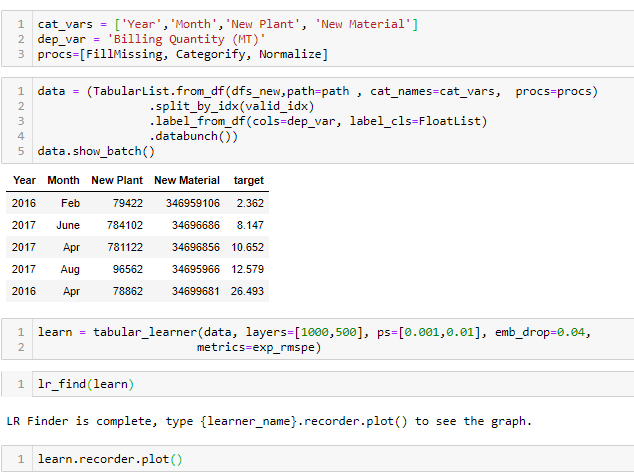# Getting higher error rate (rmse value) for tabular dataset

Hey i am trying to predict dataset similar to rossman data.
Dataset includes 5 fields and Billing quantity is my target.

The dataset is of 3 years records containing 85 New materials sales at 81 different New Plants.
Datatypes of respective fields are:
Year int64
Month object
New Plant int64
New Material int64
Billing Quantity (MT) float64
dtype: object
I have attached the databunch code and I am getting error rate as NanNew user here, adding to this conversation as I couldn’t see how to start a new thread…

I think I’ve got a similar issue to the one above - essentially I have a Tabular model that’s training well with an RMSE of ~2.0.

Before submitting to a competition, I’m using re-adding the entire training and validation set as a test set, then using get_preds against this (to ensure I’ve got the workflow functioning properly). However when I export that model, load it back in and get_preds against that test set, the RMSE is around 60!

Would really appreciate if someone could help me out, and point out where I’m going wrong - this is a massive difference. I’ve included my code and some of the differences in results below:

# Drop timestamps, rename target and declare continuous vs categorical variables for fast.ai

cleaned_df = merged_df.drop(columns=[‘target_timestamp’]).rename(columns={
‘target_PPO:AC4_1A:TIC7201-PV’: ‘target’
})

for i in range(0, len(cleaned_df.columns)):
cleaned_df.iloc[:,i] = pd.to_numeric(cleaned_df.iloc[:,i], errors=‘ignore’)

cont_vars = cleaned_df.columns.values.tolist()
cont_vars.remove(‘timestamp’)

cat_vars = []

valid_idx = range(int(.7len(cleaned_df)), int(.9len(cleaned_df)))
procs=[FillMissing, Categorify, Normalize]
dep_var = ‘target’

# Create indexed databunch

data = (TabularList.from_df(cleaned_df, path=data_path, cat_names=cat_vars, cont_names=cont_vars, procs=procs)
.split_by_idx(valid_idx)
.label_from_df(cols=dep_var, label_cls=FloatList, log=False)
.databunch())

max_y = (np.max(cleaned_df[‘target’])*1.2)
y_range = torch.tensor([0, max_y], device=defaults.device)

learn = tabular_learner(data, layers=[1000,500], ps=[0.01,0.1], emb_drop=0.04,
y_range=y_range, metrics=root_mean_squared_error)

learn.fit_one_cycle(1, 1e-4, wd=0.1)

test_df = merged_df.drop(columns=[‘timestamp’, ‘target_timestamp’,‘target_PPO:AC4_1A:TIC7201-PV’])
test_df[‘target’] = np.arange(len(test_df))

for i in range(0, len(test_df.columns)):
test_df.iloc[:,i] = pd.to_numeric(test_df.iloc[:,i], errors=‘ignore’)

model_name = ‘regressor’

test=TabularList.from_df(test_df, cat_names=cat_vars, cont_names=cont_vars))

preds, y = test_learn.get_preds(DatasetType.Test)

px = preds.numpy()
px = pd.DataFrame(px)
px

testing = pd.merge(merged_df, px, left_index=True, right_index=True)
testing[‘target_PPO:AC4_1A:TIC7201-PV’] = testing[‘target_PPO:AC4_1A:TIC7201-PV’].astype(‘float’)

# Calculate RMSE

((testing[‘target_PPO:AC4_1A:TIC7201-PV’] - testing.iloc) ** 2).mean() ** .5

# Result: 59.336321997070314

# Added for context - As you can see below, there’s quite a difference between the preds and actuals